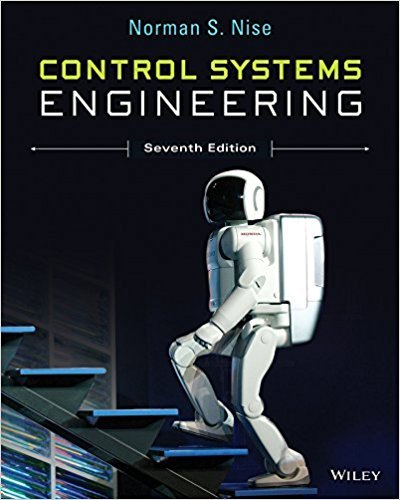×
Get Full Access to Control Systems Engineering - 7 Edition - Chapter 5 - Problem 13
Get Full Access to Control Systems Engineering - 7 Edition - Chapter 5 - Problem 13

×

# For the system shown in Figure P5.13, find the poles ofISBN: 9781118170519 162

## Solution for problem 13 Chapter 5

Control Systems Engineering | 7th Edition

• Textbook Solutions
• 2901 Step-by-step solutions solved by professors and subject experts
• Get 24/7 help from StudySoup virtual teaching assistantsControl Systems Engineering | 7th Edition

4 5 1 418 Reviews
21
4
Problem 13

For the system shown in Figure P5.13, find the poles of the closed-loop transfer function, Ts Cs=Rs. [Section: 5.3]

Step-by-Step Solution:
Step 1 of 3

Basic Structures: Sets, Functions, Sequences, & Sums Introduction • Sets are one of the basic building blocks for the types of objects considered in discrete mathematics. • Important for counting. • Programming languages have set operations. • Set theory is an important branch of mathematics. • Many different systems of axioms have been used to develop set theory. • Here we are not concerned with a formal set of axioms for set theory. Instead, we will use what is called naïve set theory. Sets • A set is an unordered collection of objects. • the students in this class • the chairs in this room • The objects in a set are called the elements, or members of the set. A set is said to contain its elements. • The notation a ∈ A denotes that a is an

Step 2 of 3

Step 3 of 3

##### ISBN: 9781118170519

Unlock Textbook Solution